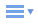﻿ PHP foreach - 網頁設計教學站# PHP foreach

PHP foreach 提供陣列很便利的輸出方式，用起來與 for 迴圈while 迴圈有點類似，不同的在於處理陣列更加的容易，且 foreach 僅用於處理陣列，如果用來處理其他不同的數據組或變數，很可能會出現錯誤情況，foreach 有兩種使用結構。

PHP foreach 語法結構
foreach ( \$array as \$value )
... 執行程式碼 ...

foreach ( \$array as \$key => \$value )
... 執行程式碼 ...

PHP foreach 輸出範例
<?php
foreach (array(1, 2, 3, 4) as \$value) {
echo 'value='.\$value.'<br>';
}
echo '<br>';
foreach (array(1, 2, 3, 4) as \$key => \$value) {
echo 'key='.\$key.' and value='.\$value.'<br>';
}
?>

value=1
value=2
value=3
value=4

key=0 and value=1
key=1 and value=2
key=2 and value=3
key=3 and value=4